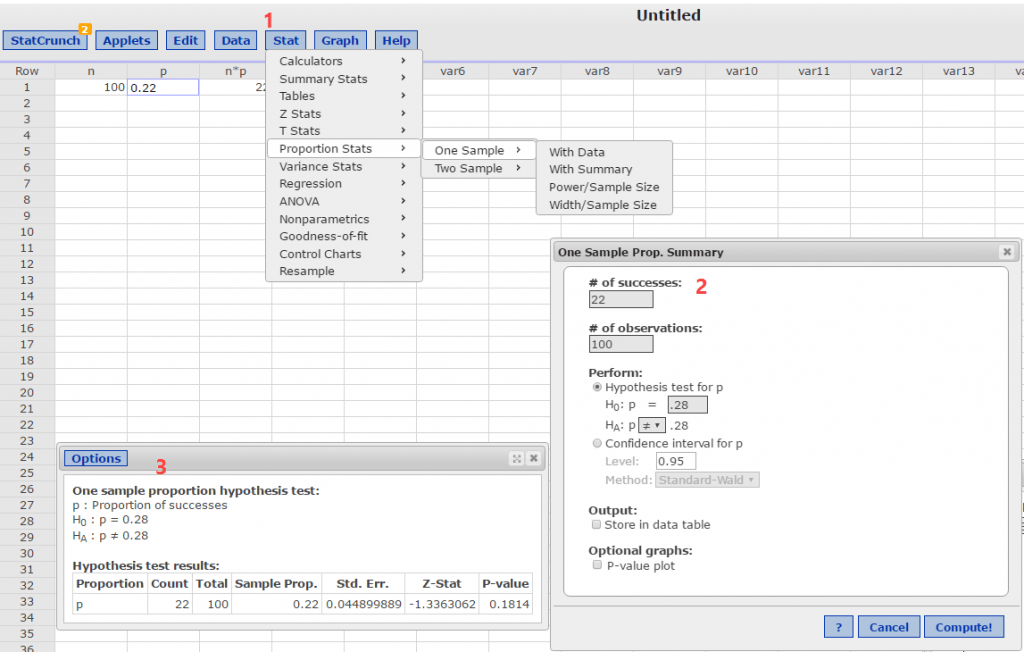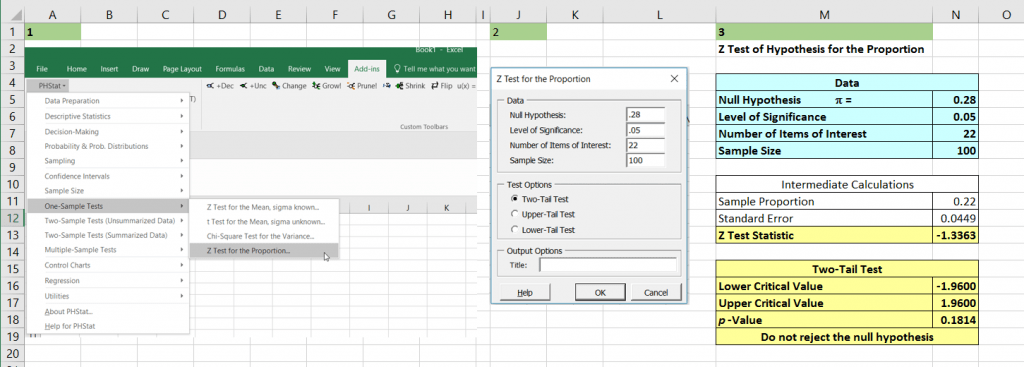# No Standard Deviation? How do I get the standardized test statistic?

I get this question a lot in my BUS 233 Business Statistics course. It comes when students see a problem similar to the following in their homework or on a quiz:

In a sample of 1000 home buyers, you find that 457 home buyers found their real estate agent through a friend. At α=0.08, can you reject the claim that 50% of home buyers find their real estate agent through a friend?
a) Write the claim mathematically and identify H0 and Ha. (b) Find the critical value(s) and identify the rejection region(s). (c) Find the standardized test statistic. (d) Decide whether to reject or fail to reject the null hypothesis.

If you run into a problem which asks you to find the standardized test statistic but does not give you the standard deviation, it is probably a proportion problem and this one is just that.

Solution:

Ho: p = 0.50; Ha: p ≠ 0.50.

Using the z-test for proportion p, the population proportion. [This is a binomial distribution problem because it is essentially a Yes/No {win/lose or success/failure} answer and the probability, which equals p, remains constant.]

1. Check that n*p and n*q are both >= 5. Recall q = 1- [note: if either np or nq are < 5, use the binomial experiment approach.] In this example n*p = 500 and n*q = 500, so we can use the z-test.
2. Find the test statistic which is the sample proportion,. In this example,= 0.457 which is observed count/n or 457/1000.
3. Find the standardized test statistic:1. Compare the standardized test statistic with the critical value of z or find the p-value using technology.

You can solve the hypothesis test for proportion quickly using StatCrunch or Excel/PHStat:

StatCrunch solution for similar proportion problem.

1. Find the critical value(s) of z using the Stat > Calculators > Normal command sequence using alpha or alpha/2 depending upon the tail of the test.
2. Use the command sequence Stat > Proportion Stats > One Sample > With summary.Excel/PHStat solution in three steps:

1. Use command sequence Add-ins > PHStat > One Sample Tests > Z Test for Proportion.
2. Fill in the pop-up dialog box and click OK.
3. Answers and supporting intermediate calculations appear. Note: PHStat gives you the critical value(s) of z.This site uses Akismet to reduce spam. Learn how your comment data is processed.